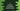# C program to calculate simple interest# C program to find out simple interest :

In this C programming tutorial, we will learn how to write a program to find out the simple interest. The user will enter the input values and the program will calculate and print out the result to the user.

## How to calculate simple interest :

To calculate simple interest, we need the principal amount, i.e. the amount where we will calculate the interest, time and rate of interest. We can write down the simple interest formulae as below :

``Simple Interest = (N * P * R)/100``

Where, N = Time for the interest P = Principal amount R = Rate of interest

So, to calculate the simple interest using a C program, we need to get these three values from the user.

## Algorithm :

The following algorithm we will use to calculate the simple interest :

1. Ask the user to enter the principal amount (P).
2. Ask the user to enter the time for the interest (N).
3. Ask the user to enter the rate of interest (R).
4. Calculate the simple interest and print out the result.

## C program :

Now, let’s try to write down the c program :

``````#include<stdio.h>

int main(){
//1
int n,p,r,simpleInterest;

//2
printf("Enter principal amount : ");
scanf("%d",&p);

//3
printf("Enter rate of interest : ");
scanf("%d",&r);

//4
printf("Enter number of year : ");
scanf("%d",&n);

//5
simpleInterest = (n * p * r)/100;

//6
printf("Simple Interest is : %d\n",simpleInterest);
}``````

### Explanation :

The commented numbers in the above program denote the step numbers below :

1. Create four integer variables n,p,r, and simpleInterest to hold the number of years,principal amount,rate of interest and simple interest respectively.
2. Ask the user to enter the principal amount. Read the value using scanf and store it in the variable p.
3. Ask the user to enter the rate of interest. Read and store it in variable r similarly.
4. Similarly, read the number of years and store it in n.
5. Calculate the simple interest using the above formulae we have discussed and store it in ‘simpleInterest’ variable.
6. Finally, print out the simple interest we have calculated on the above step.

### Conclusion :

This tutorial has shown you how to calculate the simple interest in C using user provided inputs. Try to run the program we have shown above and drop one comment below if you have any queries or questions.

You might also like :